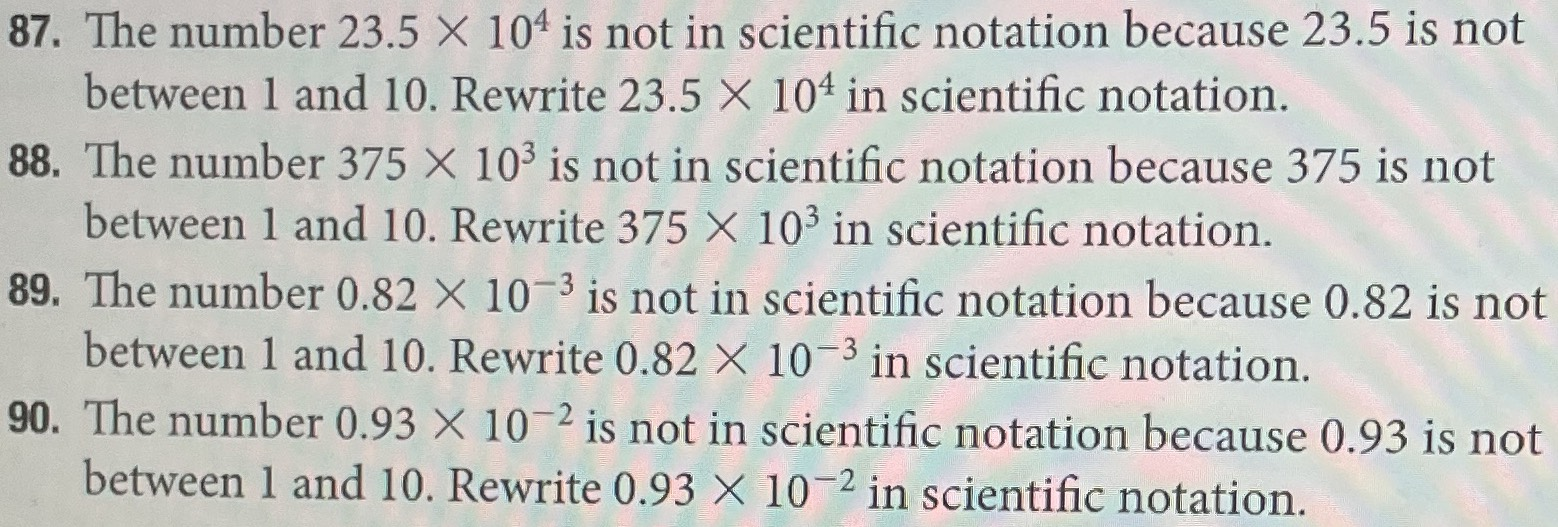### ¿Todavía tienes preguntas de matemáticas?

Pregunte a nuestros tutores expertos
Algebra
Pregunta87. The number $$23.5 \times 10 ^ { 4 }$$ is not in scientific notation because $$23.5$$ is not between $$1$$ and $$10$$ . Rewrite $$23.5 \times 10 ^ { 4 }$$ in scientific notation.

88. The number $$375 \times 10 ^ { 3 }$$ is not in scientific notation because $$375$$ is not between $$1$$ and $$10$$ . Rewrite $$375 \times 10 ^ { 3 }$$ in scientific notation.

89. The number $$0.82 \times 10 ^ { - 3 }$$ is not in scientific notation because $$0.82$$ is not between $$1$$ and $$10$$ . Rewrite $$0.82 \times 10 ^ { - 3 }$$ in scientific notation.

90. The number $$0.93 \times 10 ^ { - 2 }$$ is not in scientific notation because $$0.93$$ is not between $$1$$ and $$10$$ . Rewrite $$0.93 \times 10 ^ { - 2 }$$ in scientific notation.

87. $$23.5 \times 10^4 = 2.35 \times 10^5$$

88. $$375 \times 10^3 = 3.75 \times 10^5$$

89. $$0.82 \times 10^{- 3} = 8.2 \times 10^{- 4}$$

90. $$0.93 \times 10^{- 2} = 9.3 \times 10^{- 3}$$

Solución
View full explanation on CameraMath App.